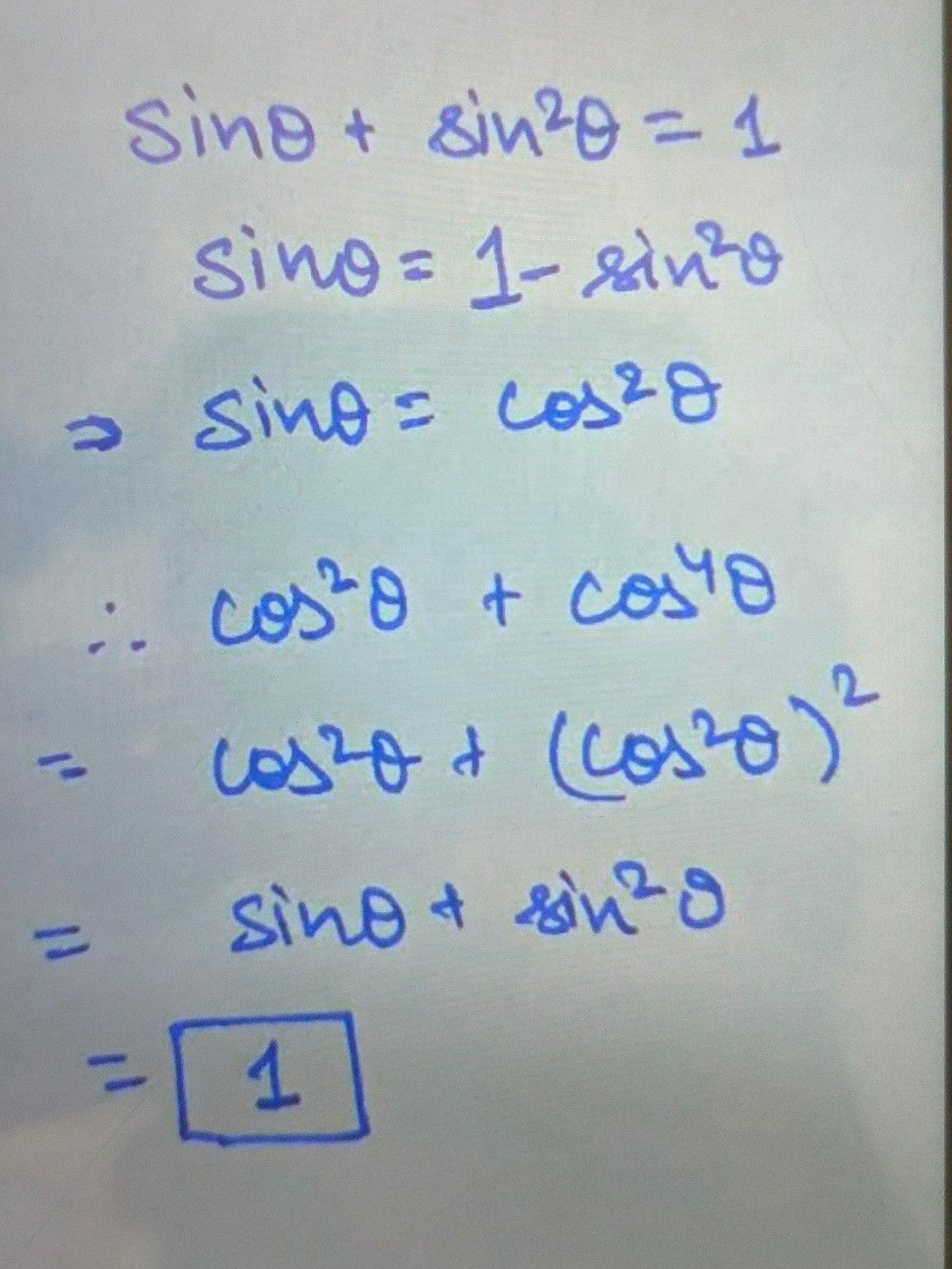Symbol
Problem$=1$ If $sin$ theta $+sin^{n}2theta=$ $+cos^{n}4theta=$ Prove that cos^2theta $+cos^{n}4t$
Calculus
Search count: 117
SolutionQanda teacher - priyanka